# KSEEB Solutions for Class 9 Maths Chapter 8 Heron’s Formula Ex 8.2

In this chapter, we provide KSEEB SSLC Class 9 Maths Chapter 8 Heron’s Formula Ex 8.2 for English medium students, Which will very helpful for every student in their exams. Students can download the latest KSEEB SSLC Class 9 Maths Chapter 8 Heron’s Formula Ex 8.2 pdf, free KSEEB SSLC Class 9 Maths Chapter 8 Heron’s Formula Ex 8.2 pdf download. Now you will get step by step solution to each question.

## Karnataka Board Class 9 Maths Chapter 8 Heron’s Formula Ex 8.2

Question 1.
A park, in the shape of a quadrilateral ABCD, has ∠C = 90°, AB = 9 m, BC = 12 m, CD = 5 m and AD = 8 m. How much area does it occupy?
Solution:
BD is joined.
In Right angled ABCD, ∠C = 90°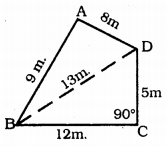As per Pythagoras Theorem,
BD2 = BC2 + CD2
= (12)2 + (5)2
= 144 + 25
BD2 = 169
∴ BD = 13m.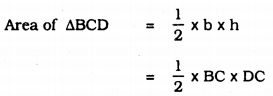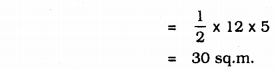Now, in ∆ABD,
Let a = 9m, b = 8m, c = 13m.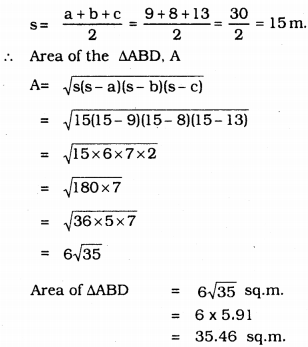= Area(∆ABD) + Area (∆BCD)
= 35.46 + 30
= 65.46 sq.m.
Area of quadrilateral ABCD = 65.46 sq.m.

Question 2.
Find the area of a quadrilateral ABCD which AB = 3 cm, BC = 4 cm, CD = 4 cm, DA = 5 cm, and AC = 5 cm.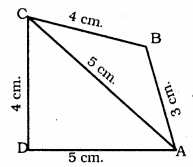Solution:
In quadrilateral ABCD, AC is the diagonal.
In ∆ABC, a = 5cm, b = 3 cm, c = 4 cm.
∴ Perimeter = a + b + c = 5 + 3 + 4 = 12 cm.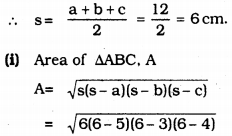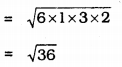∴ Area(∆ABC) = 6 sq. cm.
In ∆ADC, a1 = 5cm, b1 = 5 cm, c1 = 4cm.
∴ Perimeter = a1 + b1 + c1
= 5 + 5 + 4 = 14 cm.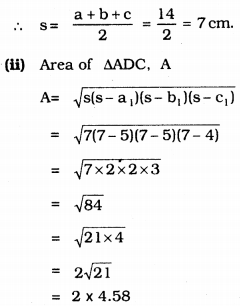Area of ∆ADC = 9.16 sq.cm.
= 6 + 9.16
= 15.16 sq. cm.

Question 3.
Radha made a picture of an aeroplane with coloured paper as shows in Fig. Find the total area of the paper used.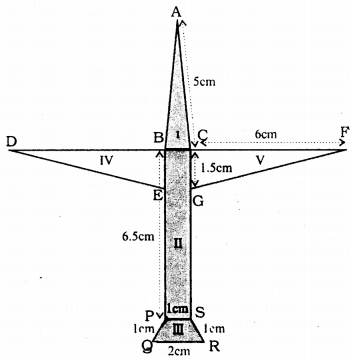Solution:
(I) ∆ABC is an isosceles triangle.
AB = AC = 5 cm, and BC = 1 cm.
∴ a = 5 cm, b = 5 cm, c = 1 cm.
∴ Perimeter = a + b + c = 5 + 5 + 1 = 11 cm.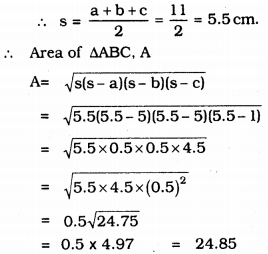∴ Area(∆ABC) = 24.85 sq. cm.

(II) PBCS is a rectangle.
l = 6.5 cm, b = 1 cm.
Area of rectangle PBCS = l x× b
= 6.5 × 1 = 6.5 sq.cm.

(III) PQRS is a trapezium PT ⊥ QR
In Right angle ∆PTV,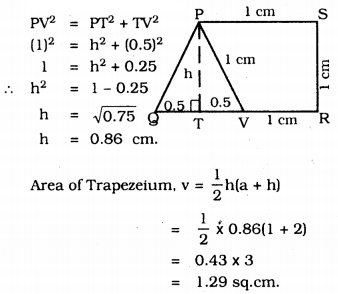(IV) Area of ADBE = Area of ∆FCG

(V)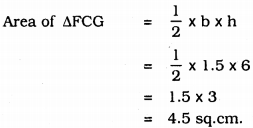∴ The total area of the paper used to make an aeroplane
= 2.485 + 6.5 + 1.29 + 4.5 + 4.5
= 19.275 sq. cm.

Question 4.
A triangle and a parallelogram have the same base and the same area. If the sides of the triangle are 26 cm, 28 cm and 30 cm, and the parallelogram stands on the base 28 cm, find the height of the parallelogram.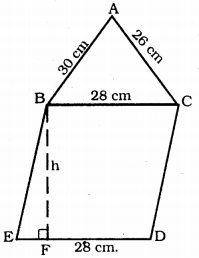Solution:
Perimeter of ∆ABC = 26 + 28 + 30 = 84 cm.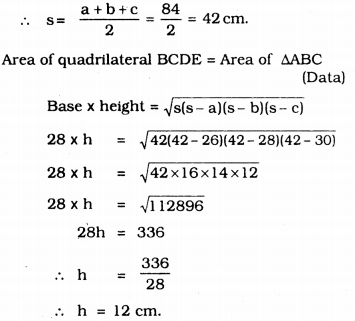Question 5.
A rhombus shaped field has green grass for 18 cows to graze. If each side of the rhombus is 30 m and its longer diagonal is 48 m, how much area of grass field will each cow be getting ?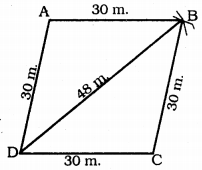Solution:
ABCD is a rhombus.
Area(∆ABD) = Area(∆BDC).
In ∆ABD, a = 30 m, b = 30 m, c = 48 m.
∴ Perimeter= a + b + c = 30 + 30 + 48 = 108 m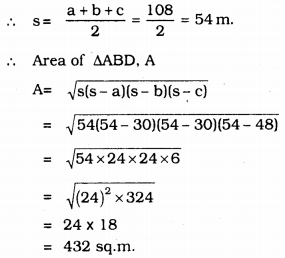But, Area of whole field = 2 × area of ∆ABD
= 2 × 432
= 864 sq.m.
∴ Are required for growing grass for 18 cows is 864 sq.m.
∴ Area required for growing grass for 1 cow ………. ??
86418 = 48 sq.m.

Question 6.
An umbrella is made by stitching 10 triangular pieces of cloth of two different colours, each piece measuring 20 cm, 50 cm and 50 cm. How much cloth of each colour is required for the umbrella?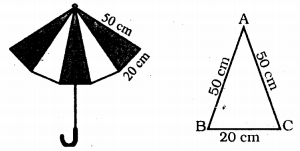Solution:
Each sides of triangular shaped cloth
Let a = 50 cm, b = 50 cm, c = 20 cm.
∴ Perimeter = a + b + c
= 50 + 50 + 20 = 120 cm.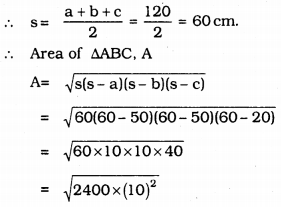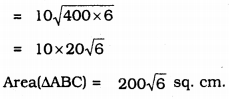∴ Total Area of Umbrella mad up with 10 triangular shaped cloth is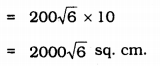Question 7.
A kite in the shape of a square with a diagonal 32 cm and an isosceles triangle of base 8 cm and sides 6 cm each is to be made of three different shades as shown in Fig. How much paper of each shade has been used in it ?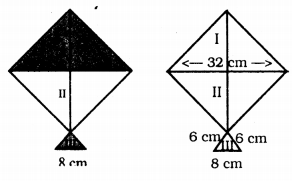Solution:
Kite is in the shape of square.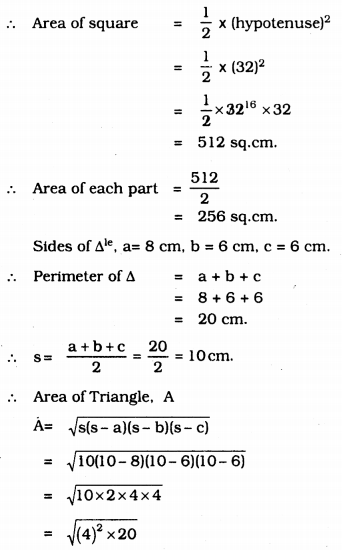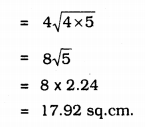∴ Total area = I + II + III
= 256 + 256 + 17.92
= 549.92 sq.cm.

Question 8.
A floral design of a floor is made up of 16 tiles which are triangular, the sides of the triangle being 9 cm, 28 cm and 35 cm. Find the cost of polishing the tiles at the rate of 50p per cm2.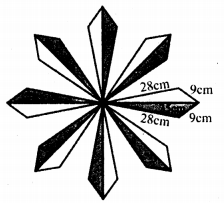Solution:
In the given figure there are 16 triangles.
Each sides of triangle are
a = 28 cm, b = 9 cm, c = 35 cm.
Perimeter of triangle = a + b + c
= 28 + 9 + 35 = 72 cm.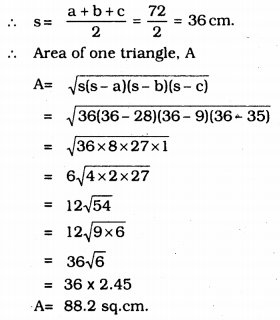∴ Area of 16 triangles = 16 × 88.2 = 1411.2 sq.cm.
Polish expenditure for 1 sq.cm. = 50 p = Re. 0.5.
∴ Polish expenditure for 1411.2sq. cm. =?
= 1411.2 × 0.5
= Rs 705.6

Question 9.
A field is in the shape of a trapezium whose parallel sides are 25 m and 10 m. The non-parallel sides are 14 m and 13 m. Find the area of the field.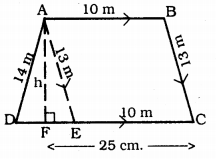Solution:
ABCD is a trapezium.
AB || DC.
Draw AE || BC and AF ⊥ DC.
∴ ABCE is a parallelogram.
DE = DC – EC = 25 – 10
DE = 15 cm.
a = 14 cm, b = 13 cm. c = 15 cm.
∴ Perimeter= a + b + c
= 14 + 13 + 15 = 42 cm.
∴ s = a+b+c2=422=21cm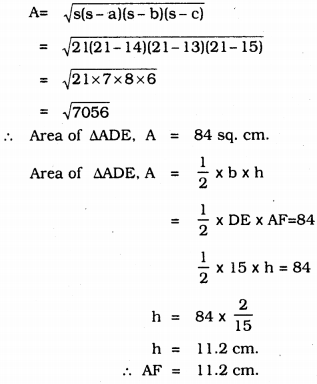(ii) Area of quadrilateral ABCE = base × height
= EC × AF
= 10 × 11.2
= 112.0
= 112 sq.cm.
∴ Complete area of trapezium ABCD, A
= 84 + 112
= 196 sq.cm.

All Chapter KSEEB Solutions For Class 9 Maths

—————————————————————————–

All Subject KSEEB Solutions For Class 9

*************************************************

I think you got complete solutions for this chapter. If You have any queries regarding this chapter, please comment on the below section our subject teacher will answer you. We tried our best to give complete solutions so you got good marks in your exam.

If these solutions have helped you, you can also share kseebsolutionsfor.com to your friends.

Best of Luck!!